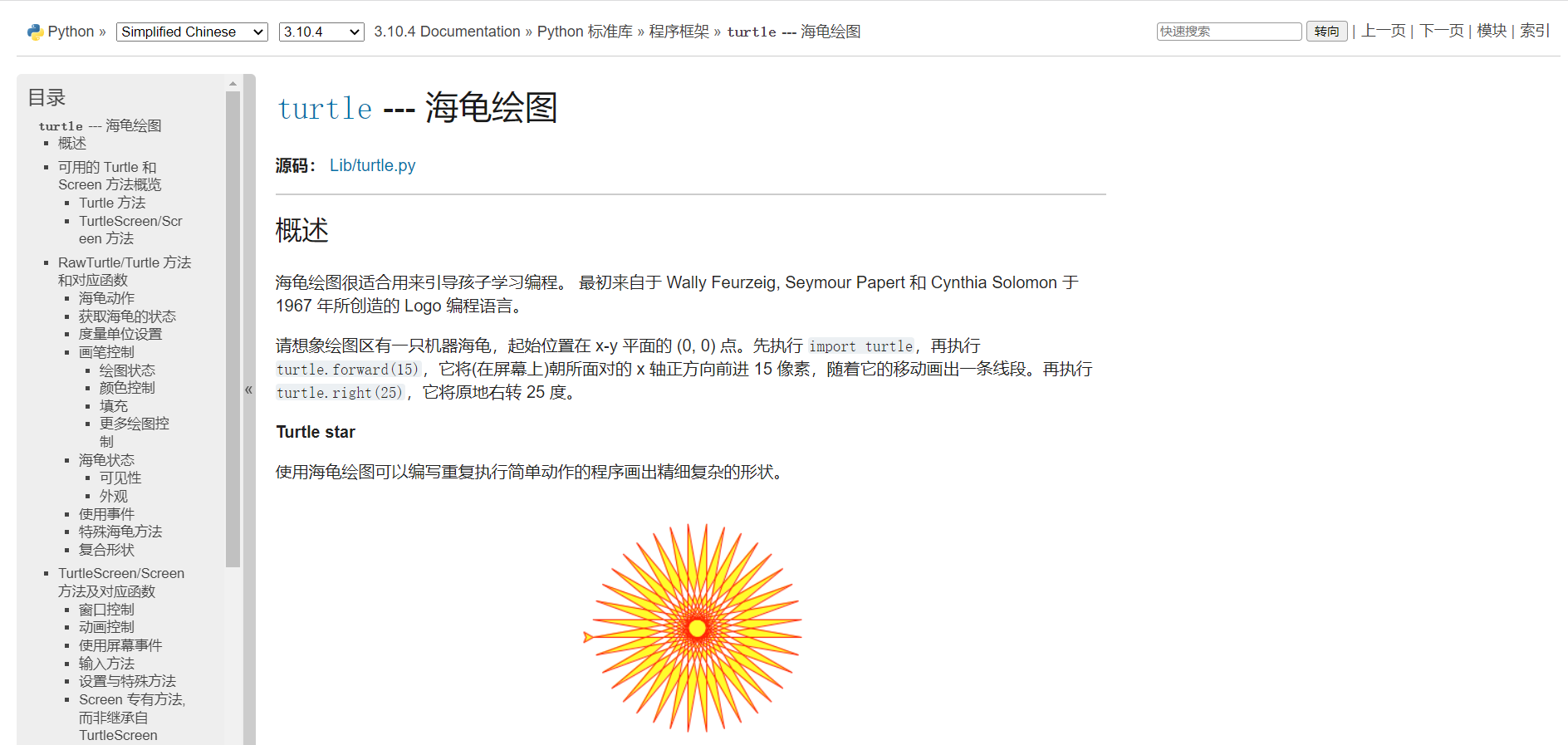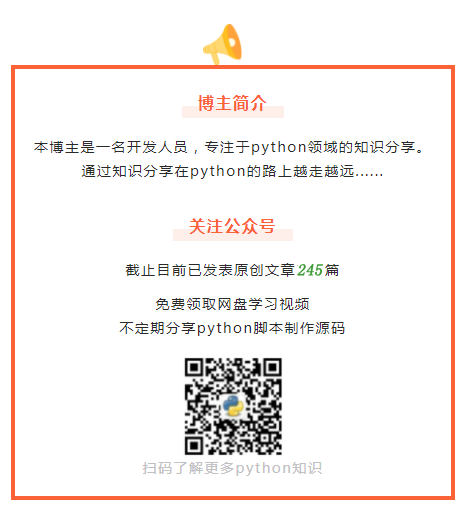# python中最简单的turtle绘图：奥运五环

https://docs.python.org/zh-cn/3/library/tle.htmlimport turtle as tle


def init():
tle.pensize(20)

init()


def draw_cricle(circle_size=100,x=-250,y=-30,color='blue'):
tle.penup()
tle.goto(x, y)
tle.pendown()
tle.color(color)
tle.circle(100)


draw_cricle(x=-250,y=-30,color='blue')
draw_cricle(x=0,y=-30,color='black')
draw_cricle(x=250,y=-30,color='red')
draw_cricle(x=-125,y=-105,color='yellow')
draw_cricle(x=125,y=-105,color='green')


screen = tle.Screen()
screen.exitonclick()【往期精彩】

python多线程同步售票系统解决思路...

python四个性能检测工具，包括函数的运行内存、时间等等...

python-turtle绘制雪容融，已打包成exe可直接运行（附源码）

posted @ 2022-05-10 20:55  Python集中营  阅读(152)  评论(0编辑  收藏  举报